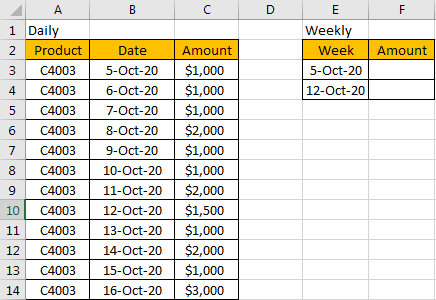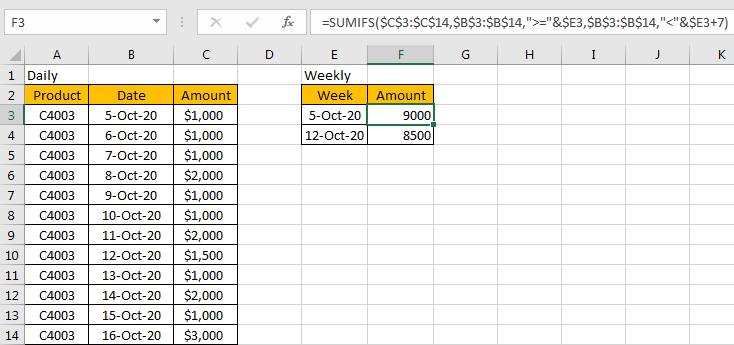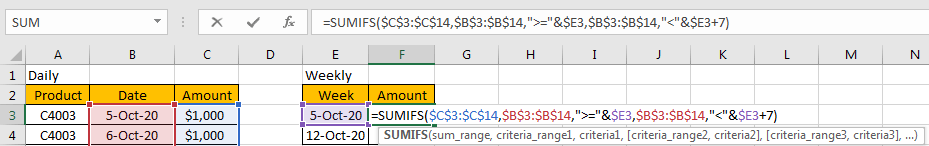# How to Sum by Week in Excel

At the end of work on Friday, we often summarize data based on daily report and create a weekly report, so sometimes we need to sum values by week for summary. Like sum data by month, we can use a formula contains SUMIFS function to sum data by week properly. This tutorial will show you the way with simple descriptions and screenshots, and let you know how to use SUMIFS function to sum data per your requirement clearly.

EXAMPLE:FORMULA:

In F3, enter the formula below:

=SUMIFS(\$C\$3:\$C\$14,\$B\$3:\$B\$14,”>=”&\$E3,\$B\$3:\$B\$14,”<“&\$E3+7)

HOW THIS FOMULA WORKS:

This formula is based on SUMIFS function. For SUMIFS function, it sum data with multiple criteria, the syntax is =SUMIFS(sum_range, criteria_range1, criteria1, [criteria_range2, criteria2], …).

In this case, \$C\$3:\$C\$14 is the sum range, it provides matching values for sum, as it is an absolute range, so we add \$ before row and column number, so when copying formula to other cells, this range is fixed.

\$B\$3:\$B\$14 is the criteria range, it provides all dates, it is an absolute range in this case as well, so we add \$ before row and column number; as we want to find out date from this range belongs to week of E3 (date 5-Oct-20), so we must find out date greater than or equals to E3,so we enter ‘\$B\$3:\$B\$14,”>=”&\$E3’ here. We add \$ before E to lock E column, so even row is changed when coping the formula for other date in E column, the column is locked at that time.

Unlike sum data by month, the start date and end date are not fixed for weeks, so if we want to sum data by week, we must make sure the first date is Monday in the formula. In this instance, 5-Oct-20 is Monday, and a week has 7 days, so just use E3+7 as then end of the week. So, we enter \$B\$3:\$B\$14,”<“&\$E3+7 as another criteria.

RESULT:

Let’s see if this formula works correctly.NOTES:

1.If you are consumed about the range in the formula, you can name range firstly, for example

\$C\$3:\$C\$14=Amount;

\$B\$3:\$B\$14=Date;

Then update formula to =SUMIFS(Amount,Date,”>=”&\$E3,Date,”<“&\$E3+7). It looks clearly now.2. Make sure the date is Monday in Week column. If you want to set Friday as date in Week column, you can use \$E3-5 as the start of week, \$E3 as the end of week.

Update formula to =SUMIFS(\$C\$3:\$C\$14,\$B\$3:\$B\$14,”>=”&\$E3-5,\$B\$3:\$B\$14,”<=”&\$E3). See example below:### Related Functions

• Excel SUMIFS Function
The Excel SUMIFS function sum the numbers in the range of cells that meet a single or multiple criteria that you specify. The syntax of the SUMIFS function is as below:=SUMIFS (sum_range, criteria_range1, criteria1, [criteria_range2, criteria2], …)…